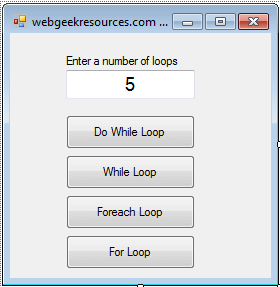# Loops in C#

This tutorial in c# will demonstrate the four types of loop; do while loop, while loop, foreach loop and for loop.

This is a gui program (graphical user interface) with windows form, buttons and other graphical controls.

Let’s start doing the program.

1. Open your Microsoft Visual Studio or Visual C# 2010.
2. Select File and create a New Project.
3. Select Visual C#, then Windows Form Application (for application with a windows form user interface).
4. Sample layout of the form, this will serve only as a guide, feel free to design your form.5. Based on the form above, we need the following controls:
1. 1 label
2. 1 textbox
3. 4 buttons
6. We will now code for the Do While Loop, double click the Do While Loop button and paste the code below.
```int myNumber=0;
do
{
MessageBox.Show("number: " + myNumber,"do while loop");
myNumber = myNumber + 1;
} while (myNumber < int.Parse(TextBox1.Text));
```

Code Explanation:

The do while loop will execute the code block once, then the loop will continue until the expression returns false. The code to be executed in this code is the displaying of message box and incrementing of our variable myNumber.

Code to execute (code block):

```MessageBox.Show("number: " + myNumber,"do while loop");
myNumber = myNumber + 1;
```

Expression statement:

```myNumber < int.Parse(TextBox1.Text)
```

the code block will continue to execute if the expression statement is still true, it will only terminate if the statement returns false value.

1. Code for the While Loop, double click the While Loop button and paste the code below.
```int myNumber = 0;
while (myNumber < int.Parse(TextBox1.Text))
{
MessageBox.Show("number: " + myNumber, "while loop");
myNumber = myNumber + 1;
}
```

Code Explanation:

While loop will first evaluate the statement before executing the statements, if the expression is false then the loop will never start. The code blocks will only continue to execute as long as the expression is true, it is terminated if the expression is false.

1. Code for Foreach Loop, double click the Foreach button and paste the code below.
```int[] array1 = { 0, 1, 2, 3, 4, 5 };
foreach (int n in array1)
{
MessageBox.Show("number: " + n, "foreach loop");
```

}
Code Explanation
:

According to the Visual Studio MSDN Library, The foreach statement is used to iterate through the collection to get the information that you want, but cannot be used to add or remove items from the source collection to avoid unpredictable side effects. This is an example of displaying the contents (integers) in an array.

1. For Loop, double click the For Loop button and paste the code below.
```for (int i = 0; i < int.Parse(TextBox1.Text); i++)
MessageBox.Show("number: " + i, "for loop");
```

Code Explanation:

The for loop will execute the block of codes for as long as the condition is true. The for loop will initialize first the variable then the condition will be evaluated or tested, if the condition is false then the loop will terminate. The loop will start if the test condition is true. A counter variable will be updated before the loop will continue.

Note: when you double click the button control the default event is the Click Event, which means that the code will be triggered when the users will click the button.

Sample code:

```private void button5_Click(object sender, EventArgs e)
{
// paste your code here
}
```
1. Don’t forget to save the project.

Download